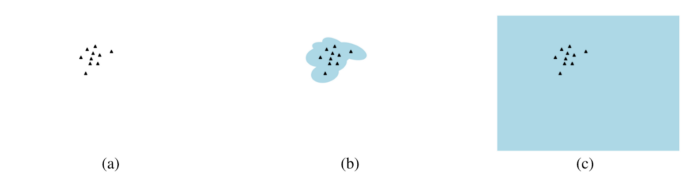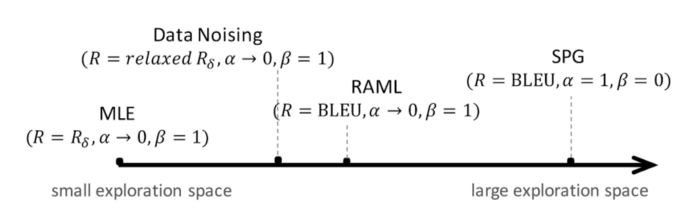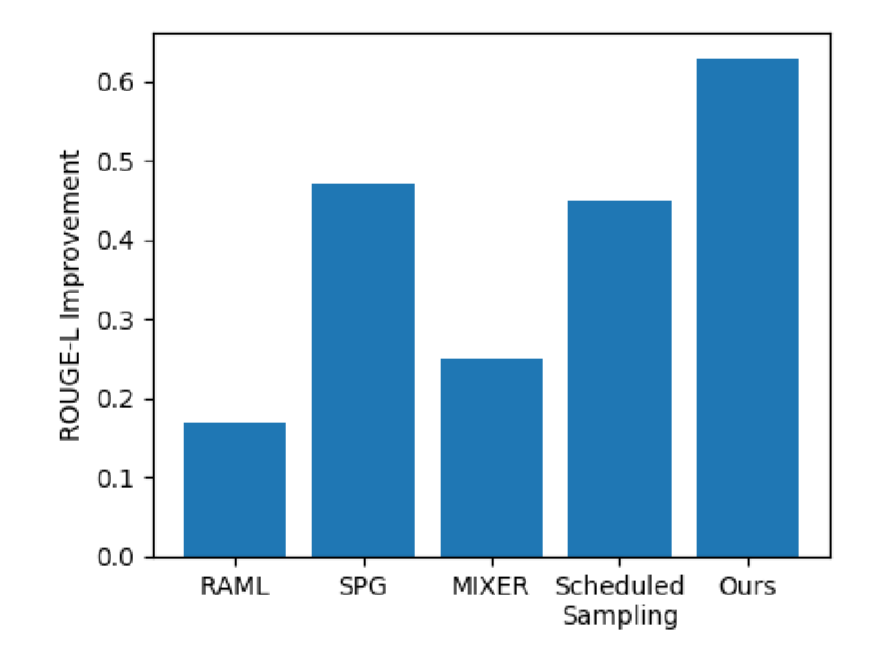November 27, 20188

# Connecting The Dots Between MLE & RL For Sequence GenerationCrossposted on the Texar blog.

Sequence generation is a ubiquitous problem in many applications, such as machine translation, text summarization, image captioning, and so forth.

Recently, we published a paper on a unified perspective of a variety of well-used learning algorithms for sequence generation, based on a generalized entropy regularized policy optimization formulation. We show that these algorithms are mathematically equivalent to specifying certain hyperparameter configurations in the framework. The new principled treatment provides systematic understanding and comparison among the algorithms and inspires further enhancement. We also propose a new interpolation algorithm based on the universal framework, which shows consistent improvement in machine translation and text summarization.

The development of sequence models such as recurrent neural networks (RNNs) with diﬀerent cells and attention mechanisms has enabled great advances in tasks requiring sequence generation. These models can be trained with a variety of learning algorithms, which we’ll outline below.

## Popular Algorithms (The Dots)

The standard training algorithm is based on maximum-likelihood estimation (MLE), which seeks to maximize the log-likelihood of ground-truth sequences. Despite its computational simplicity and efﬁciency, MLE training suﬀers from exposure bias — that is, the model is trained to predict the next token given the ground-truth tokens that came before. Since the resulting model does not have access to the ground truth, at test time, the tokens generated by the model itself are used to make the next prediction instead. This discrepancy between training and testing can cause mistakes in prediction to quickly accumulate.

There have been several efforts to alleviate this issue, many of which resort to reinforcement learning (RL) techniques. For example, Ranzato et al., 2015, adopt a policy gradient algorithm that avoids the training/testing discrepancy by using the same decoding strategy at both training and test time. However, RL-based approaches for sequence generation can face prohibitively poor sample efﬁciency and high variance.

For more practical training, others have developed a diverse set of methods that are in a middle ground between the MLE and RL paradigms. For example, RAML (Norouzi et al., 2016) adds reward-aware perturbation to the MLE data examples, SPG (Ding & Soricut, 2017) leverages reward distribution for eﬀective sampling of policy gradient, and other approaches such as data noising (Xie et al., 2017) also show improved results.

Maximum Likelihood Estimation (MLE)

Maximum likelihood estimation is the most widely-used approach to train a sequence generation model due to its simplicity and eﬃciency. MLE aims to ﬁnd the optimal parameter value that maximizes the data log-likelihood:

Reward Augmented Maximum Likelihood (RAML)

RAML was originally proposed to incorporate task metric rewards into MLE training and has shown superior performance to vanilla MLE. Speciﬁcally, RAML introduces an exponentiated reward distribution e(y|y*) ∝ exp{R(y|y*)} where R, as in vanilla policy optimization, is a task metric such as BLEU. RAML maximizes the following objective:

The RAML objective reduces to the vanilla MLE objective if we replace the task reward R in e(y|y*) with the MLE δ-reward, which is a reward function defined as:

Data Noising

Adding noise to training data is a widely adopted technique for regularizing models. Previous work has proposed several data noising strategies in the sequence generation context. For example, unigram noising with probability γ replaces each token in data y* with a sample from the unigram frequency distribution. The resulting noisy data is then used in MLE training. Formally, it is equivalent to using a reward:

where u(·) is the unigram frequency distribution. With a relaxed (i.e., smoothed) reward, data noising expands the exploration space of vanilla MLE locally. The effect is essentially the same as the RAML algorithm, except that RAML expands the exploration space based on the task metric reward.

SPG was developed with the purpose of adapting the vanilla policy gradient to use as the reward for sampling. SPG has the following objective:

where R is a common reward. As a variant of the standard policy gradient algorithm, SPG aims to address the exposure bias problem and shows promising results.Figure 1. Effective exploration space of different algorithms. (a): The exploration space of MLE is exactly the set of training examples. (b): RAML and Data Noising use smooth rewards and allow larger exploration space surrounding the training examples. ©: Common policy optimization such as SPG basically allows the whole exploration space.

## Connecting the Dots

We establish a uniﬁed perspective of this broad set of learning algorithms. Speciﬁcally, we present a generalized entropy regularized policy optimization (ERPO) framework and show that the apparently diverse algorithms, such as MLE, RAML, SPG, and data noising, can all be re-formulated as special instances of the framework with the only diﬀerence being the choice of reward and the values of a couple of hyperparameters.

In addition to a new understanding of existing algorithms, our uniﬁed perspective also facilitates the development of new algorithms for improved learning. We present an example new algorithm that, as training proceeds, gradually expands the exploration space by annealing the reward and hyperparameter values. The annealing, in eﬀect, dynamically interpolates among the existing algorithms. Experiments on machine translation and text summarization show that the interpolation algorithm achieves signiﬁcant improvement over the various existing methods.

## The General Framework

Our general framework is aimed at unifying all of the above algorithms with a common mathematical formulation. The framework is based on policy optimization, which, in general, maximizes the expected reward under the model distribution. A rich line of research into entropy regularized policy optimization (ERPO) has stabilized learning by augmenting policy optimization with information theoretic regularizers. Here, we present a generalized formulation of ERPO. Specifically, assuming a variational distribution q(y|x), we adopt the objective:

where (x, y*) is the pair from training data; y is the sentence sampled following distribution q(y|x); KL(·||·) is the KL divergence; H(·) is the Shannon Entropy; α and β are balancing weights of the respective terms; and is the sequence generation model parameterized with θ.

Using the Lagrange multipliers method, this objective can be maximized with an EM-style procedure that iterates two coordinate ascent steps optimizing q and θ, respectively. At iteration n:

## Other Algorithms as Special Instances

By assuming the ERPO framework, we can characterize other sequence generation algorithms as special instances within it.

Maximum Likelihood Estimation (MLE)

Let (R = Rδ, α → 0, β = 1). From the E-step of ERPO, we have q(y|x) = 1 if y = y*, and 0 otherwise. The M-step is therefore equivalent to:

which recovers precisely the MLE objective.

That is, MLE can be seen as an instance of the policy optimization algorithm with the δ-reward and the above weight values. Any sample y that fails to match precisely the data y* will receive a negative inﬁnite reward and never contribute to model learning.

Reward Augmented Maximum Likelihood (RAML)

As we discussed, the RAML objective reduces to the vanilla MLE objective if we replace the task reward R in e(y|y*) with the MLE δ-reward. The relation between MLE and RAML still holds within ERPO. Similar to the way we recovered MLE from ERPO, if we let (α → 0, β = 1), but set R to the task metric reward, then the M-step of ERPO is precisely equivalent to maximizing the above RAML objective.

Data Noising

Though previous literature has covered techniques such as including a data pre-processing step that differs from the above learning algorithms, the ERPO framework can also subsume data noising as a special instance. Speciﬁcally, starting from the ERPO reformulation of MLE, which takes (R = Rδ, α → 0, β = 1), data noising can be formulated as using the unigram-relaxed discussed above.

SPG can also readily ﬁt into our ERPO framework. By taking the gradient of the objective of SPG w.r.t θ, we immediately get the same update rule as in ERPO with (α = 1, β = 0, R = common reward).

Note that the only difference between the SPG and RAML conﬁguration is that now α = 1. SPG thus moves a step further than RAML by leveraging both the reward and the model distribution for full exploration. Sufﬁcient exploration at training time would, in theory, boost the test-time performance. However, with the increased learning difﬁculty, additional sophisticated optimization and approximation techniques must be used (Ding & Soricut, 2017) in order to make the training practical.Figure 2. A unified formulation of different learning algorithms. Each algorithm is a special instance of the general ERPO framework taking certain specifications of the hyperparameters (R, α, β).

## Application: Interpolation Algorithm

In our generalized ERPO framework, a series of well-used learning algorithms can all be understood as instances of the framework with certain speciﬁcations of the three hyperparameters (R, α, β). Each of the algorithms can be seen as a point in the hyperparameter space (Figure 1). Generally, a point with a more restricted reward function R and a very small α tends to have a smaller effective exploration space and allow efﬁcient learning (e.g., MLE), while in contrast, a point with smooth R and a larger α would lead to a more difﬁcult learning problem, but permit more sufﬁcient exploration and better test-time performance (e.g., (softmax) policy gradient). In our paper, we also explore an example algorithm that interpolates the existing ones.

The interpolation algorithm exploits the natural idea of starting learning from the most restricted yet easiest problem conﬁguration, and gradually expands the exploration space to reduce the discrepancy from the test time — the easy-to-hard learning paradigm. As we have mapped common algorithms to points in the hyperparameter space, interpolation becomes very straightforward and only requires annealing of the hyperparameter values.

## Experimental Results

We evaluate the above interpolation algorithm on the tasks of machine translation and text summarization. The proposed algorithm consistently improves over a variety of previous methods, as shown in the figures below.Figure 3. The graph on the left is the convergence curve of different learning algorithms in the machine translation task. On the right is the improvement on text summarization in comparison to MLE.

Please check out our paper for more details on this work: https://arxiv.org/abs/1811.09740

## Code

Our code for experiments is available here. Implementations are based on Texar, a general-purpose and easy-to-use text generation toolkit.